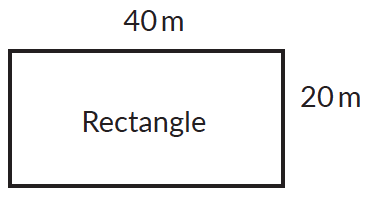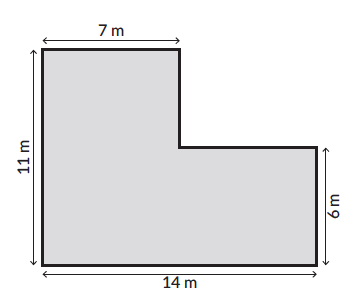In the verge of coronavirus pandemic, we are providing FREE access to our entire Online Curriculum to ensure Learning Doesn't STOP!

# Perimeter

Go back to  'Mensuration and Measurement'

## What is perimeter?

The total length of the boundary of a closed shape is called its perimeter. Let’s try and understand this using an example,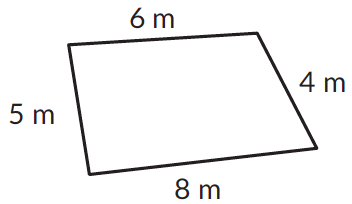Perimeter of the shape $$= 5\,m + 8\,m + 4\,m + 6\,m = 15\,m$$

## Formula for calculating perimeter of different shapes

 Shape Formula for perimeter Examples$$2\,(\text{length} + \text{breadth})$$\begin{align}P &= 2\,(4 \,cm + 2\, cm) \\ &= 2\, (6\, cm)\\ &= 12 \,cm\end{align}$$4 \times \text{side}$$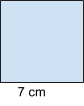\begin{align}P &= 4 \times 7 \,cm \\ & = 28 \,cm \end{align}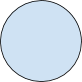\begin{align} 2 \pi r \end{align}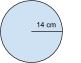\begin{align}P & = 2 \pi r \\ & = 2 \times { 22 \over 7} \times 14\\ & = 88 \,cm \end{align}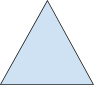$$a+b+c$$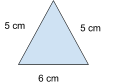\begin{align}P &= 5\,cm + 5 \,cm + 6 \,cm \\ &= 10 \,cm + 6 \,cm \\ &= 16 \,cm \end{align}

## Tips and tricks

• Tip: Children often get confused between area and perimeter as concepts. A thorough understanding can be built by asking the child to trace the surface of any 3D shape and measure its outside boundary. And then finding out the perimeter.
• While calculating the perimeter of composite shapes children often miss summing up one of the sides to avoid this ask the children to calculate the horizontal shapes first followed by the vertical shapes. In the given figure calculating the perimeter of shape 1, followed by shape 2 and then shape 3 would ensure that all the sides are accounted for.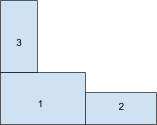• For rectilinear shapes doubling the sides doubles the perimeter. For example, the perimeter of the given shape is 2 (l + b) = 2 (40 cm + 20cm) = 120 m. However, if the sides double up (length 80m and width 40m) the perimeter will also double up, it will be 140m.